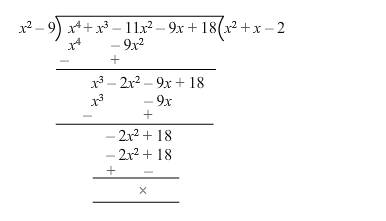# If 3 and −3 are two zeros of the polynomial

Question:

If 3 and $-3$ are two zeros of the polynomial $\left(x^{4}+x^{3}-11 x^{2}-9 x+18\right)$, find all the zeros of the given polynomial.

Solution:

Let $f(x)=x^{4}+x^{3}-11 x^{2}-9 x+18$

Since 3 and $-3$ are the zeroes of $f(x)$, it follows that each one of $(x+3)$ and

$(x-3)$ is a factor of $f(x)$

Consequently, $(x-3)(x+3)=\left(x^{2}-9\right)$ is a factor of $f(x) .$

On dividing $f(x)$ by $\left(x^{2}-9\right)$, we get:$f(x)=0=>\left(x^{2}+x-2\right)\left(x^{2}-9\right)=0$

$=>\left(x^{2}+2 x-x-2\right)(x-3)(x+3)$

$=>(x-1)(x+2)(x-3)(x+3)=0$

$=>x=1$ or $x=-2$ or $x=3$ or $x=-3$

Hence, all the zeroes are $1,-2,3$ and $-3$.